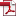• KSII Transactions on Internet and Information Systems
Monthly Online Journal (eISSN: 1976-7277)

# Analytical Approximation Algorithm for the Inverse of the Power of the Incomplete Gamma Function Based on Extreme Value Theory

Vol. 15, No. 12, December 31, 202110.3837/tiis.2021.12.017, Download Paper (Free):#### Abstract

This study proposes an analytical approximation algorithm based on extreme value theory (EVT) for the inverse of the power of the incomplete Gamma function. First, the Gumbel function is used to approximate the power of the incomplete Gamma function, and the corresponding inverse problem is transformed into the inversion of an exponential function. Then, using the tail equivalence theorem, the normalized coefficient of the general Weibull distribution function is employed to replace the normalized coefficient of the random variable following a Gamma distribution, and the approximate closed form solution is obtained. The effects of equation parameters on the algorithm performance are evaluated through simulation analysis under various conditions, and the performance of this algorithm is compared to those of the Newton iterative algorithm and other existing approximate analytical algorithms. The proposed algorithm exhibits good approximation performance under appropriate parameter settings. Finally, the performance of this method is evaluated by calculating the thresholds of space-time block coding and space-frequency block coding pattern recognition in multiple-input and multiple-output orthogonal frequency division multiplexing. The analytical approximation method can be applied to other related situations involving the maximum statistics of independent and identically distributed random variables following Gamma distributions.